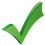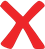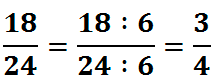# Online math exercises: Adding and Subtracting fractions

Score: Level1 5 + 2 2 = = —— —— 2 51 step – find the common denominator and the same factor used to multiply

 • 2 + • 3 Check2 step – to multiply each numerator by the same factor

 + Check denominator3 step – perform calculations (addition or subtraction)

 Check denominator4 step – if necessary then simplify the fraction and record the answer at the beginning

Victory

Congratulations, you win## Reminder

Do not forget to simplify the fraction